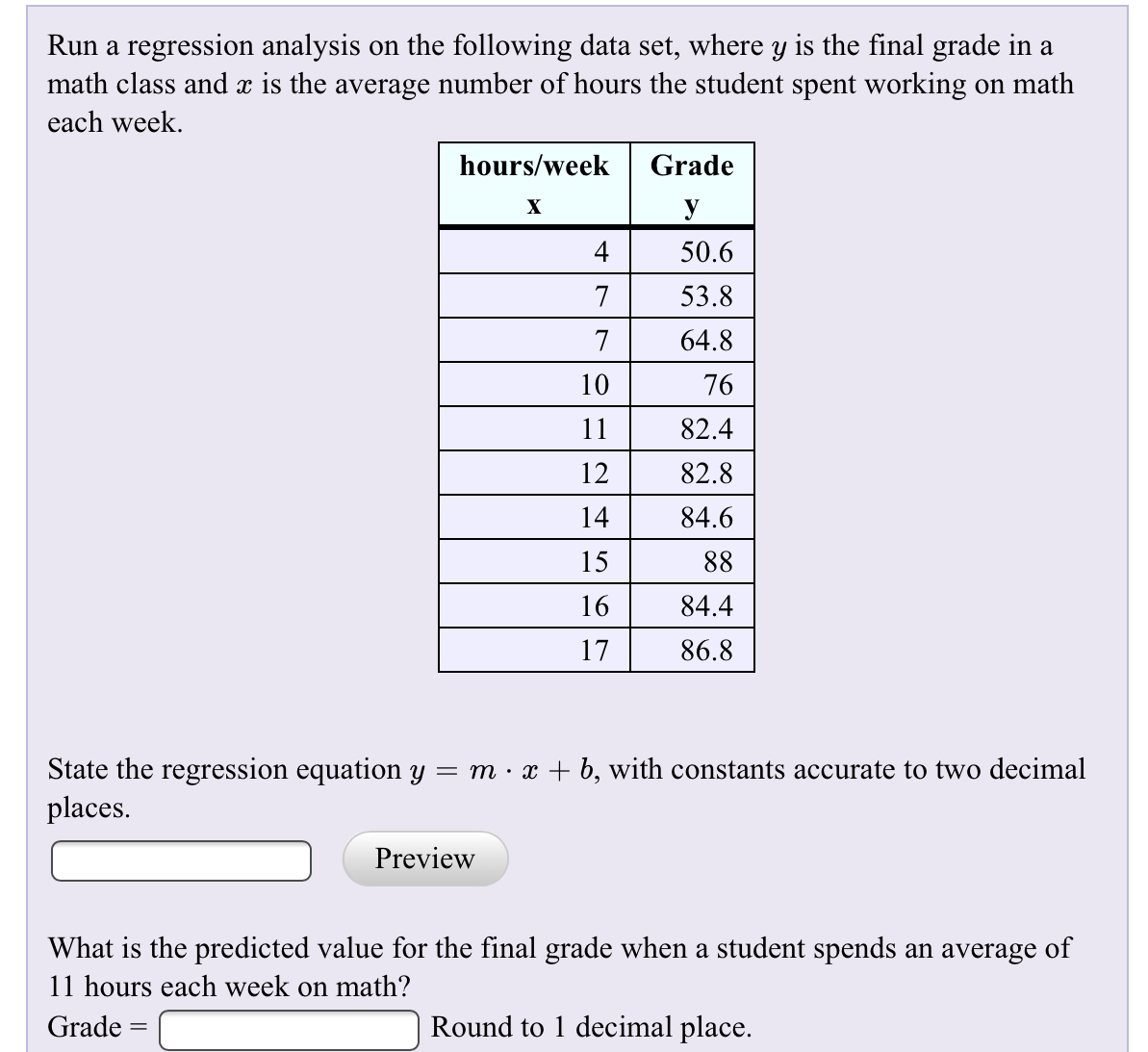# Run a regression analysis on the following data set, where y is the final grade in amath class and x is the average number of hours the student spent working on matheach weekhours/weekGradeXУ50.6453.8764.87107682.41182.81284.61415881684.41786.8State the regression equation y = m x + b, with constants accurate to two decimalplacesPreviewWhat is the predicted value for the final grade when a student spends an average of11 hours each week on math?Round to 1 decimal place.Grade

Question
119 views

How do find the answer on paper and the TI84help_outlineImage TranscriptioncloseRun a regression analysis on the following data set, where y is the final grade in a math class and x is the average number of hours the student spent working on math each week hours/week Grade X У 50.6 4 53.8 7 64.8 7 10 76 82.4 11 82.8 12 84.6 14 15 88 16 84.4 17 86.8 State the regression equation y = m x + b, with constants accurate to two decimal places Preview What is the predicted value for the final grade when a student spends an average of 11 hours each week on math? Round to 1 decimal place. Grade fullscreen
check_circle

Step 1

Computation of regression line equation:

Step-by-step procedure to find the regression line equation using TI-84 calculator:

• Click on STAT > Enter> Type data values of Hours/Week x under L1 and Grade y under L2.
• Enter STAT > CALC> 4: LinReg(ax+b)
• Choose XList as L1YLIST as L2.
• Select Calculate and Enter.

From the output of TI-84 calculator, the value of a is 2.977632362 and the value of b is 41.77275431.

a)

Thus, the regression equation is y =2.977632362x + 41...

### Want to see the full answer?

See Solution

#### Want to see this answer and more?

Solutions are written by subject experts who are available 24/7. Questions are typically answered within 1 hour.*

See Solution
*Response times may vary by subject and question.
Tagged in

### Other